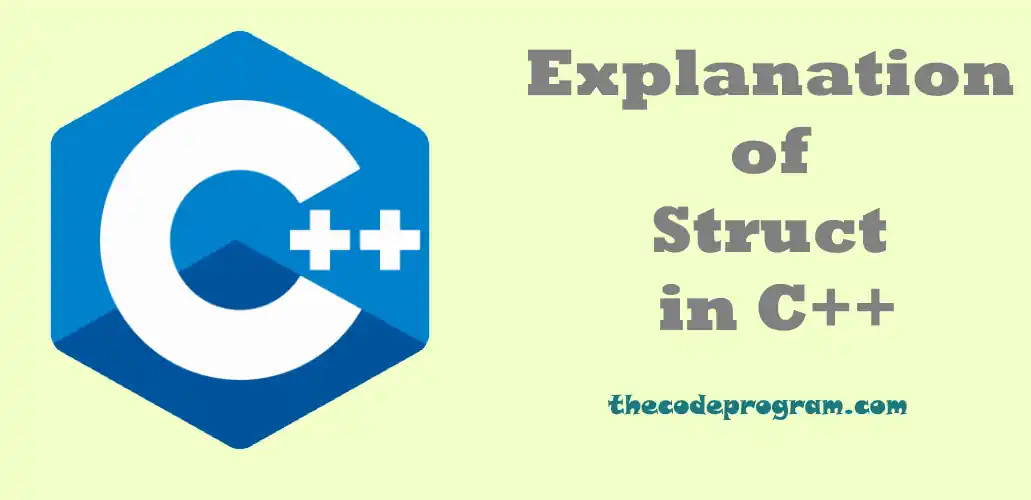## Explanation of Struct in C++

Hello everyone, in this article we are going to talk about the one of the most efficient data building blocks as alias Structs in C++ with the help of examples.

Let's get started.

### What is Struct in C++

Struct is a data building block which allows us to store and organise custom data types in C++. We can store the informations which are related with same group. Structs are also like classes, we can define methods, we can use some concepts of classes. Bigger difference between struct and classes default access modifier is public for struct and private for class.

Structs have no encapsulation concept of classes, so they are mainly using for grouping and storing data instead of perform complex operations like Classes are doing.

Below you can see an example of defining a struct
``````
using namespace std;

struct TheStruct {
// Variables
std::string name;
int year;

// Constructor
TheStruct(std::string name, int year)
: name(name), year(year) {}

// Method Definition
void method(){
// Some operations
}
};
``````

As you can see above we have defined a struct and we defined its variables. Also we added its constructor to initialise this struct with initial values and we have added some methods to this struct to perform some operations.

Now let's make some examples about how to use a struct in C++ with a real world example.

``````
#include <iostream>
using namespace std;

struct Rectangle3D {
// Define points as a variable
double x;
double y;
double z;

// Constructor
Rectangle3D(double x, double y, double z)
: x(x), y(y), z(z) {}

// Calculation method
double calculateVolume(){
return x * y * z;
}
};

int main() {
// Initialize the structs
Rectangle3D cube(7, 7, 7);
Rectangle3D rect3d(8.4, 12.5, 3.3);

// Call the methods
std::cout << "Volume of Cube is " << cube.calculateVolume() << '\n';
std::cout << "Volume of 3d Rectangle is " << rect3d.calculateVolume() << '\n';

return 0;
}
``````
Program output will be like below
``````
Volume of Cube is 343
Volume of 3d Rectangle is 346.5
``````

As you can see above we have used a Struct like it is a class with all public variables. We initialised with initial parameters with the help of constructor and we invoked a method of the struct to perform some calculations.

We can also initialize a struct without constructur and we can set the variables after initialising. Also we can send it to a method as a parameter. Below you can see the an example about accessing the variables of a struct and sending it to a variable.
``````
#include <iostream>
using namespace std;

struct Rectangle3D {
// Define points as a variable
double x;
double y;
double z;
};

double calculateVolume(Rectangle3D rect){
return rect.x * rect.y * rect.z;
}

int main() {
// Initialize the struct
Rectangle3D rectangle;
// Set the variables
rectangle.x = 8;
rectangle.y = 3.4;
rectangle.z = 98.1;

// Invoke method with struct parameter
double volume = calculateVolume(rectangle);
std::cout << "Volume of Cube is " << volume << '\n'; // 2668.32

return 0;
}
``````

As you can see above we only defined the variables in struct and we accessed and organized the variables from outside of struct. Then we have sent it as a parameter for calculation operation.

#### Conclusion

Structs are very efficient way for storing and organising the data which is a custom type. It is very easy to use and helpful to make your code more maintainable. If your informations are small and no need to hide from outside you can consider to use structs in C++.

That is all for structs article.

Burak Hamdi TUFAN

22/11/2015

14/09/2020

13/07/2021